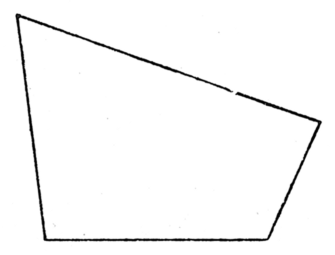#Quadrilaterals

Math Lair Home > Topics > Quadrilaterals

A quadrilateral is a four-sided figure. Several types of quadrilaterals have special names:A square is a quadrilateral with four equal sides and four equal (right) angles.A rectangle is a quadrilateral with four equal (right) angles.A rhombus is a quadrilateral with four equal sides.A parallelogram is a quadrilateral with two pairs of parallel sides.A trapezoid or a trapezium is a quadrilateral with one pair of parallel sides.There are other quadrilaterals for which none of the above definitions apply.

It can be seen from the definitions that all squares are rectangles and rhombuses, and all rhombuses and rectangles are parallelograms. The reverse is not necessarily true, however.